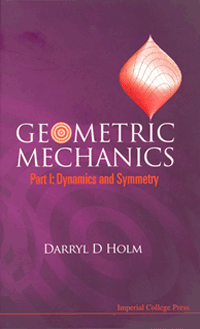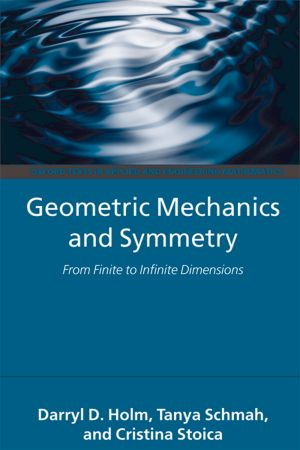# Books## Dynamics and Symmetry

This textbook introduces the tools and language of modern geometric mechanics to advanced undergraduate and beginning graduate students in mathematics, physics and engineering. It treats the dynamics of ray optics, resonant oscillators and the elastic spherical pendulum from a unified geometric viewpoint, by formulating their solutions using reduction by Lie-group symmetries. The only prerequisites are linear algebra, calculus and some familiarity with the Euler-Lagrange variational principles and canonical Poisson brackets in classical mechanics at the beginning undergraduate level.

The ideas and concepts of geometric mechanics are explained in the context of explicit examples. Through these examples, the student develops skills in performing computational manipulations, starting from Fermat's principle, working through the theory of differential forms on manifolds and transferring these ideas to the applications of reduction by symmetry to reveal Lie-Poisson Hamiltonian formulations and momentum maps in physical applications.

The many Exercises and Worked Answers aid the student to grasp the essential aspects of the subject. In addition, the modern language and application of differential forms is explained in the context of geometric mechanics, so that the importance of Lie derivatives and their flows is clear. All theorems are stated and proved explicitly.

The book's many worked exercises make it ideal for both classroom use and self-study. In particular, a substantial appendix containing both introductory examples and enhanced coursework problems with worked answers is included to help the student develop proficiency in using the powerful methods of geometric mechanics.

Contents: Fermat's Ray Optics; Newton, Lagrange and Hamilton; Differential Forms; Resonances and S1 Reduction; Elastic Spherical Pendulum; Maxwell-Bloch Equations.## Rotating, Translating and Rolling

This textbook introduces the tools and language of modern geometric mechanics to advanced undergraduate and beginning graduate students in mathematics, physics, and engineering. It treats the dynamics of rotating, spinning and rolling rigid bodies from a geometric viewpoint, by formulating their solutions as coadjoint motions generated by Lie groups. The only prerequisites are linear algebra, multivariable calculus and some familiarity with Euler-Lagrange variational principles and canonical Poisson brackets in classical mechanics at the beginning undergraduate level.

Variational calculus on tangent spaces of Lie groups is explained in the context of familiar concrete examples. Through these examples, the student develops skills in performing computational manipulations, starting from vectors and matrices, working through the theory of quaternions to understand rotations, and then transferring these skills to the computation of more abstract adjoint and coadjoint motions, Lie?Poisson Hamiltonian formulations, momentum maps and finally dynamics with nonholonomic constraints.

The 120 Exercises and 55 Worked Answers help the student to grasp the essential aspects of the subject, and to develop proficiency in using the powerful methods of geometric mechanics. In addition, all theorems are stated and proved explicitly. The book?s many examples and worked exercises make it ideal for both classroom use and self-study.

Contents: Galileo; Newton, Lagrange, Hamilton; Quaternions; Quaternionic Conjugacy; Special Orthogonal Group; The Special Euclidean Group; Geometric Mechanics on SE(3); Heavy Top Equations; The Euler-Poincare Theorem; Lie-Poisson Hamiltonian Form; Momentum Maps; Round Rolling Rigid Bodies.## Geometric Mechanics and Symmetry

Classical mechanics, one of the oldest branches of science, has undergone a long evolution, developing hand in hand with many areas of mathematics, including calculus, differential geometry, and the theory of Lie groups and Lie algebras. The modern formulations of Lagrangian and Hamiltonian mechanics, in the coordinate-free language of differential geometry, are elegant and general. They provide a unifying framework for many seemingly disparate physical systems, such as n-particle systems, rigid bodies, fluids and other continua, and electromagnetic and quantum systems.

Geometric Mechanics and Symmetry is a friendly and fast-paced introduction to the geometric approach to classical mechanics, suitable for a one- or two- semester course for beginning graduate students or advanced undergraduates. It fills a gap between traditional classical mechanics texts and advanced modern mathematical treatments of the subject. After a summary of the necessary elements of calculus on smooth manifolds and basic Lie group theory, the main body of the text considers how symmetry reduction of Hamilton's principle allows one to derive and analyze the Euler-Poincare equations for dynamics on Lie groups. Additional topics deal with rigid and pseudo-rigid bodies, the heavy top, shallow water waves, geophysical fluid dynamics and computational anatomy. The text ends with a discussion of the semidirect-product Euler-Poincare reduction theorem for ideal fluid dynamics. A variety of examples and figures illustrate the material, while the many exercises, both solved and unsolved, make the book a valuable class text.

The book provides a very comprehensive presentation of ideas and methods from geometric mechanics, aimed at the graduate-student level, but it could also be of interest for specialists who want to refresh their knowledge in this modern, elegant and unifying formulation of Lagangrian and Hamiltonian mechanics. (Jean-Francois Ganghoffer, Journal of Geometry and Symmetry in Physics)